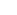## [阿里DIN] 从论文源码学习 之 embedding层如何自动更新

[阿里DIN] 从论文源码学习 之 embedding层如何自动更新

## 0x00 摘要

Deep Interest Network（DIN）是阿里妈妈精准定向检索及基础算法团队在2017年6月提出的。其针对电子商务领域（e-commerce industry）的CTR预估，重点在于充分利用/挖掘用户历史行为数据中的信息。

## 0x01 DIN源码

### 1.1 问题

```self.uid_embeddings_var = tf.get_variable("uid_embedding_var", [n_uid, EMBEDDING_DIM])
self.mid_embeddings_var = tf.get_variable("mid_embedding_var", [n_mid, EMBEDDING_DIM])
self.cat_embeddings_var = tf.get_variable("cat_embedding_var", [n_cat, EMBEDDING_DIM])```

### 1.2 答案

DIN中对应代码如下：

```# 优化更新（自动求导）
......
# 通过 session.run 进行调用更新
def train(self, sess, inps):
if self.use_negsampling:
loss, accuracy, aux_loss, _ = sess.run([self.loss, self.accuracy, self.aux_loss, self.optimizer], feed_dict={
self.uid_batch_ph: inps,
self.mid_batch_ph: inps,
self.cat_batch_ph: inps,
self.mid_his_batch_ph: inps,
self.cat_his_batch_ph: inps,
self.target_ph: inps,
self.seq_len_ph: inps,
self.lr: inps,
self.noclk_mid_batch_ph: inps,
self.noclk_cat_batch_ph: inps,
})
return loss, accuracy, aux_loss
else:
loss, accuracy, _ = sess.run([self.loss, self.accuracy, self.optimizer], feed_dict={
self.uid_batch_ph: inps,
self.mid_batch_ph: inps,
self.cat_batch_ph: inps,
self.mid_his_batch_ph: inps,
self.cat_his_batch_ph: inps,
self.target_ph: inps,
self.seq_len_ph: inps,
self.lr: inps,
})
return loss, accuracy, 0```

## 0x02 原理

### 2.1 随机梯度下降SGD

1. 抽取训练样本 x 和对应目标y 组成的数据批量。

1. 在 x 上运行网络，得到预测值 y_pred。

1. 计算网络在这批数据上的损失，用于衡量 y_pred 和 y 之间的距离。

1. 计算损失相对于网络参数的梯度［一次反向传播（backward pass）］。

1. 将参数沿着梯度的反方向移动一点，比如

`W -= step * gradient`

1. ，从而使这批数据

1. 上的损失减小一点。

### 2.3 自动求导

TensorFlow也是如此。

TensorFlow 提供的是声明式的编程接口，用户不需要关心求导的细节，只需要定义好模型得到一个loss方程，然后使用TensorFlow实现的各种Optimizer来进行运算即可。

#### 因此TensorFlow的求导，实际上是先提供每一个op求导的数学实现，然后使用链式法则求出整个表达式的导数。

```@ops.RegisterGradient("Abs")
x = op.inputs

```class RegisterGradient(object):
def __init__(self, op_type):
if not isinstance(op_type, six.string_types):
raise TypeError("op_type must be a string")
self._op_type = op_type
def __call__(self, f):
"""Registers the function `f` as gradient function for `op_type`."""
return f```

## 0x03 优化器

`minimize()`

```ctr_loss = - tf.reduce_mean(tf.log(self.y_hat) * self.target_ph)
self.loss = ctr_loss
if self.use_negsampling:
self.loss += self.aux_loss

### 3.1 Optimizer基类

slot系列 : 输入变量和name，得到的是一个 trainable=False的变量，用来记录optimizer中的中间值，比如在Momentum中，记录momentum。

Optimizer 基类的这个方法为每个实现子类预留了 `_create_slots()``_prepare()``_apply_dense()``_apply_sparse()` 四个接口出来，后面新构建的 Optimizer 只需要重写或者扩展 Optimizer 类的某几个函数即可;

### 3.2 反向传播过程

```compute_gradients()

```def minimize(self, loss, global_step=None, var_list=None,
aggregation_method=aggregation_method,

vars_with_grad = [v for g, v in grads_and_vars if g is not None]

loss : 需要被优化的Tensor
val_list : Optional list or tuple of `tf.Variable` to update to minimize `loss` . Defaults to the list of variables collected in the graph under the key `GraphKeys.TRAINABLE_VARIABLES` .```def compute_gradients(self, loss, var_list=None,
aggregation_method=None,
self._assert_valid_dtypes([loss])
if var_list is None:
var_list = (
variables.trainable_variables() +
ops.get_collection(ops.GraphKeys.TRAINABLE_RESOURCE_VARIABLES))
else:
var_list = nest.flatten(var_list)

var_list += ops.get_collection(ops.GraphKeys._STREAMING_MODEL_PORTS)
processors = [_get_processor(v) for v in var_list]
var_refs = [p.target() for p in processors]

aggregation_method=aggregation_method,

gradients 的实际定义在 `tensorflow/python/ops/gradients_impl.py` 中。把整个求导过程抽象成一个 ys=f(xs) 的函数。

xs 就是 var_list 里面输入的变量列表（在这个过程中其实这里存的是每个变量对应过来在计算图中的 op）。

`grad_ys` 存储计算出的梯度；
`gate_gradients` 是一个布尔变量，指示所有梯度是否在使用前被算出，如果设为 `True` ，可以避免竞争条件；

```queue

```def gradients(ys,
xs,
aggregation_method=None,
to_ops = [t.op for t in ys]
from_ops = [t.op for t in xs]

# Initialize queue with to_ops.
queue = collections.deque()
# Add the ops in 'to_ops' into the queue.
to_ops_set = set()

for op in to_ops:
if ready and op._id not in to_ops_set:
queue.append(op)

while queue:
# generate gradient subgraph for op.
op = queue.popleft()
if loop_state:
if loop_state:

if has_out_grads and (op._id not in stop_ops):
if is_func_call:
func_call = ops.get_default_graph()._get_function(op.type)
else:
try:

t_in.dtype != dtypes.resource):
try:

if loop_state:

ops.control_dependencies()函数用来控制计算流图的，给图中的某些节点指定计算的顺序;

```def apply_gradients(self, grads_and_vars, global_step=None, name=None):
if g is not None:
# Convert the grad to Tensor or IndexedSlices if necessary.
g = ops.convert_to_tensor_or_indexed_slices(g)
p = _get_processor(v)
var_list = [v for g, v, _ in converted_grads_and_vars if g is not None]
with ops.control_dependencies(None):
self._create_slots([_get_variable_for(v) for v in var_list])
update_ops = []
with ops.name_scope(name, self._name) as name:
self._prepare()
continue
scope_name = var.op.name if context.in_graph_mode() else ""
with ops.name_scope("update_" + scope_name), ops.colocate_with(var):
if global_step is None:
else:
with ops.control_dependencies([self._finish(update_ops, "update")]):
with ops.colocate_with(global_step):
train_op = ops.get_collection_ref(ops.GraphKeys.TRAIN_OP)

`概率论中矩的含义是：如果一个随机变量 X 服从某个分布，X 的一阶矩是 E(X)，也就是样本平均值，X 的二阶矩就是 E(X^2)，也就是样本平方的平均值。`

#### 3.3.1 _prepare

`_prepare` 函数中通过 `convert_to_tensor` 方法来存储了输入参数的 Tensor 版本。

```def _prepare(self):
self._lr_t = ops.convert_to_tensor(self._lr, name="learning_rate")
self._beta1_t = ops.convert_to_tensor(self._beta1, name="beta1")
self._beta2_t = ops.convert_to_tensor(self._beta2, name="beta2")
self._epsilon_t = ops.convert_to_tensor(self._epsilon, name="epsilon")```

#### 3.3.2 _create_slots

`_create_slots` 函数用来创建参数，比如 _beta1_power，_beta2_power

```def _create_slots(self, var_list):
first_var = min(var_list, key=lambda x: x.name)
create_new = self._beta1_power is None
if not create_new and context.in_graph_mode():
create_new = (self._beta1_power.graph is not first_var.graph)
if create_new:
with ops.colocate_with(first_var):
self._beta1_power = variable_scope.variable(self._beta1,
name="beta1_power",
trainable=False)
self._beta2_power = variable_scope.variable(self._beta2,
name="beta2_power",
trainable=False)
# Create slots for the first and second moments.
for v in var_list:
self._zeros_slot(v, "m", self._name)
self._zeros_slot(v, "v", self._name)```

`_apply_sparse_shared` 函数，首先获取所需要的参数值并存储到变量里，接着按照 Adam 算法的流程，首先计算学习率，接着计算两个 Momentum ，由于是稀疏 tensor 的更新，所以在算出更新值之后要使用 `scatter_add` 来完成加法操作， 最后将 `var_update``m_t``v_t` 的更新操作放进 `control_flow_ops.group` 中。

## 0x04 Session.run

fetches 是 run 方法的一个输入参数，这个参数可以是很多种形式的数据，run 最后的 返回值也会和 fetches 有相同的结构。

## 0xFF 参考

TensorFlow SyncReplicasOptimizer 解读

tensorflow中有向图（计算图、Graph）、上下文环境（Session）和执行流程

TensorFlow 拆包（一）：Session.Run ()

TensorFlow 源码大坑(2) Session

tensorflow源码分析（五）session.run()

TensorFlow中Session、Graph、Operation以及Tensor详解

TensorFlow 拆包（二）：TF 的数据流模型实现以及自动求导

TensorFlow实战系列3–实现反向传播

Optimizer in Tensorflow

tensorflow optimizer源码阅读笔记

tensorflow学习（三）

Tensorflow 是如何求导的？

TensorFlow的自动求导具体是在哪部分代码里实现的？

TensorFlow SyncReplicasOptimizer 解读